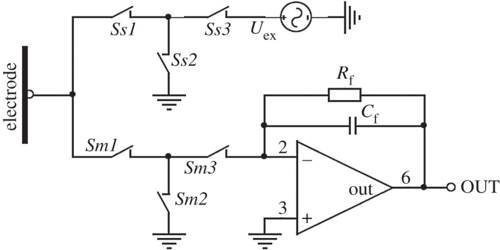# Electric Capacitance

Electric capacitance

We know, all substances have the capacity to absorb heat. It is called capacity to absorb heat or thermal capacity. The amount of heat needed to raise the temperature of a body by 1 degree is called its capacity to absorb heat. All bodies do not have the same capacity to absorb heat. It rather depends on the mass and material of the body.

Similarly, all substances have a fixed electric capacity. Generally, it is called electric capacitance or simply capacitance.

We know when charges are increased in a body its potential increases. Charge and potential are proportional to each other.

Let the potential of a body be V due to the addition of charge Q in it. So we can write,

Q ∞ V

or, Q = constant x V

or, Q = CV … …. …. (1)

Here, C is proportionality constant. This constant is the capacitance of conductor.

In language, we can define capacitance as follows,

Suppose V = 1 unit. So from equation (1) we get, Q = C.

Definition: The amount of charge needed to increase the potential of a body by 1 unit is called its electric capacitance. It is denoted by C. It depends on the size nature of the medium and presence of other bodies.

Electrical conductance of a conductor is defined as the capacity to store charge in it. Whenever the charge is applied to an insulator its potential is raised to some certain level. The charge on a conductor and its electric potential are both directly proportional to each other. So, as we increase the charge electric potential also increases.

Work and potential both are scalar quantities. So capacitance is also a scalar quantity.

Unit of capacitance: S. I. or M. K. S. unit of capacitance is Farad; in honor of famous scientist Michael Farad, this unit has been introduced. In short, it is denoted by F.

1 Farad: If 1 coulomb of charge is needed to increase the potential of a conductor by 1 V, then its capacitance is called 1 Farad.

We know, C = Q/V

so, 1F = 1C/1V

But for practical purpose Farad is a very large unit, so, smaller units are used. These are called micro-farad (μF) or, micro-micro-Farad (μμF) or, pico-farad (pF).

1F = 106 μF= 1012 μμF or, pF

or, 1 μF = 10-6 F

and 1µµF or, 1 pF = 10-12 F.